Question

# Circuit : Custom up/down counter P3.    Count Sequence          Generate a random sequence of 8 distinct...

Circuit : Custom up/down counter

P3.    Count Sequence

Generate a random sequence of 8 distinct numbers between 0 and 7.

Start at any number, and not “in order”,13576420

Document your count sequence in the Lab Notebook.

P4.    State Diagram

Create an FSM state diagram to cycle through your count sequence.

Implement forward and backward counting, based on X (1 = forward, 0 = backward).

Include a diagram. Create it with software or very neatly draw and scan.

P5.          D flip-flop design (review for final)

Determine the state table. Recognize that the Aldec Wizard will use regular binary encoding.

Determine equations for flip flop inputs and circuit outputs.

Please write FSM, state table and equations for FF input and circuit outputs.

the state table and state graph is shown below: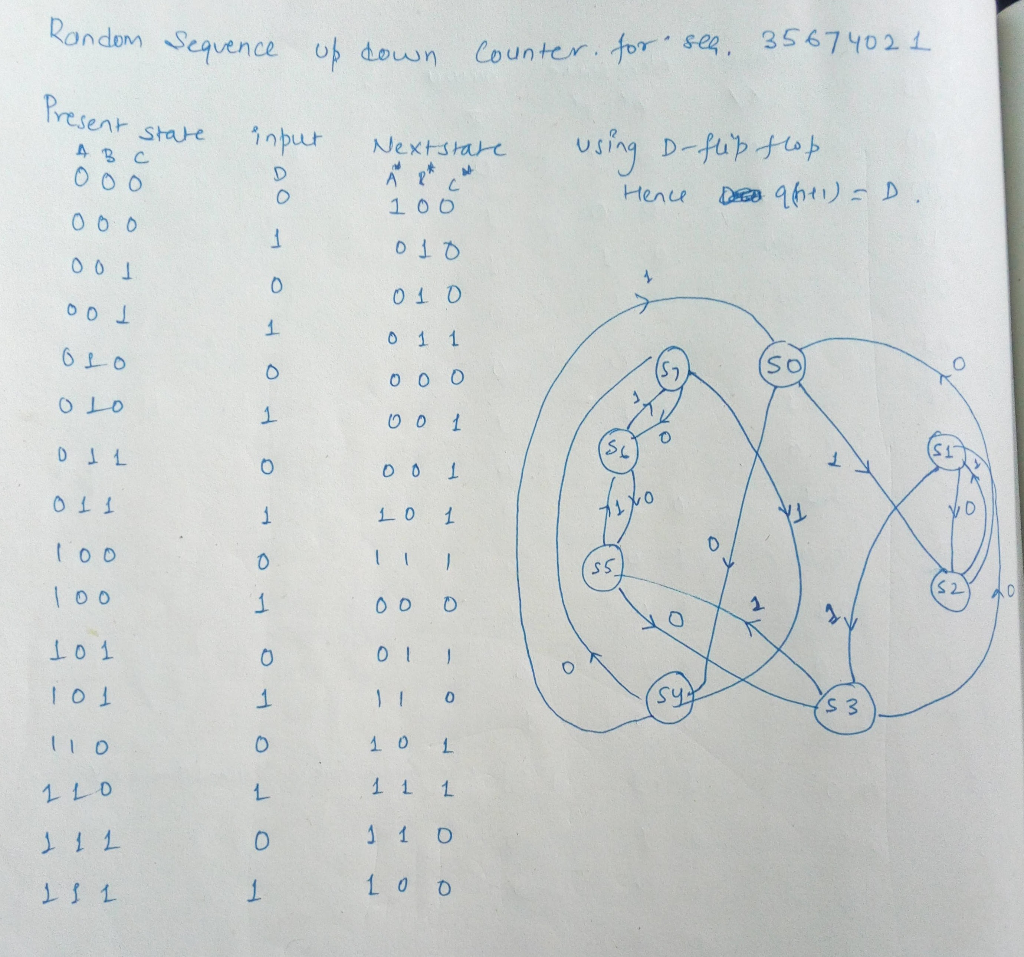k maps and next state equations:

d0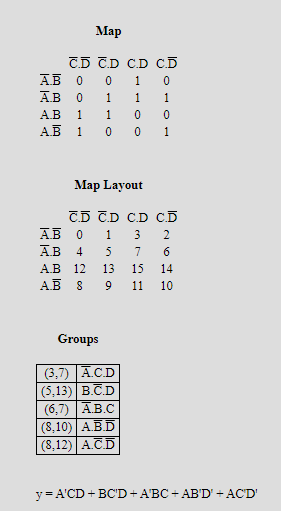for D1: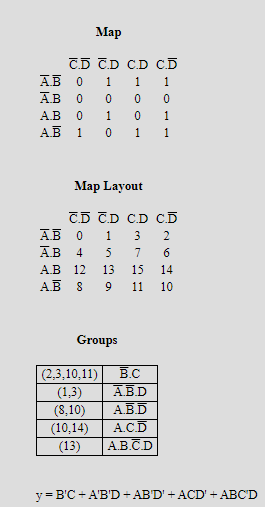for D2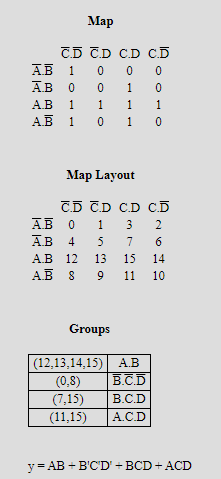the output of the circuits is Z2=A

Z1=B

Z0=C

#### Earn Coins

Coins can be redeemed for fabulous gifts.

Similar Homework Help Questions
• ### Design a 3-bit down counter FSM with no inputs and three outputs. Do this using a...

Design a 3-bit down counter FSM with no inputs and three outputs. Do this using a T flip flop. a. Draw a state diagram and the corresponding state table. b. Derive the equations for output functions and flip-flop input functions c. Draw the logic circuit diagram

• ### Instructor: Dr. A,Sctt 3. 130 pts. totall A "marching I's counter" outputs the following sequence in...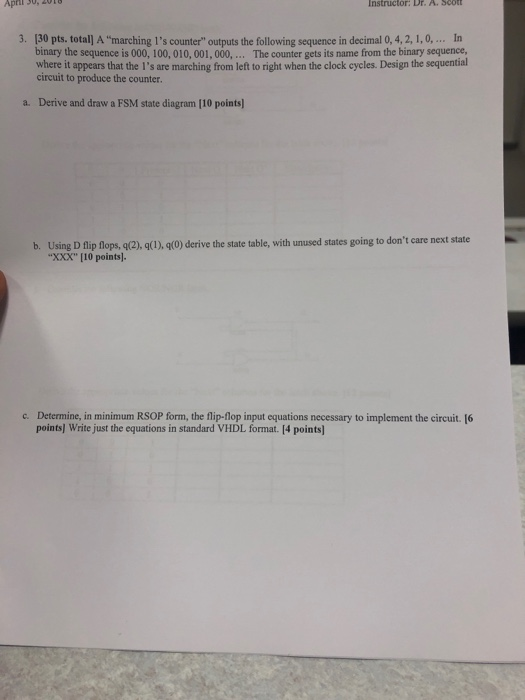Instructor: Dr. A,Sctt 3. 130 pts. totall A "marching I's counter" outputs the following sequence in decimal 0, 4,2, 1,0,... In The counter gets its name from the binary sequence, binary the sequence is 000, 100, 010, 001, 000, where it appears that the I's are marching from left to right when the clock cycles. Design the sequential circuit to produce the counter. Derive and draw a FSM state diagram [10 points) a. b. Using D fip lops,(), g(1), (o)...

• ### 1) Design a synchronous 3-bit binary UP/DOWN counter uses the following counting pattern 10.2.3.7.6.40.1.3...) the counter...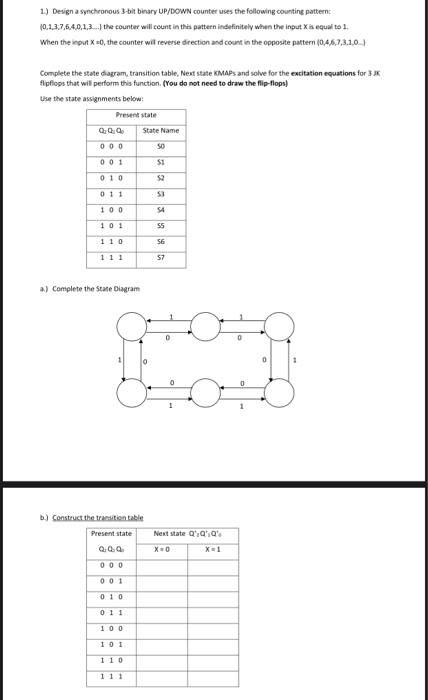1) Design a synchronous 3-bit binary UP/DOWN counter uses the following counting pattern 10.2.3.7.6.40.1.3...) the counter will count in this pattern indefinitely when the input X is equal to 1. When the input the counter will reverse direction and count in the opposite pattern 0. 4 7310) Complete the state diagram, transition table, New state s and solve for the recitation equations for flipflops that will perform this function. (You do not need to draw the flip-flops Use the state...

• ### (20 points) Using any state encodings you want, generate a state table for the following state diagram. Note that there...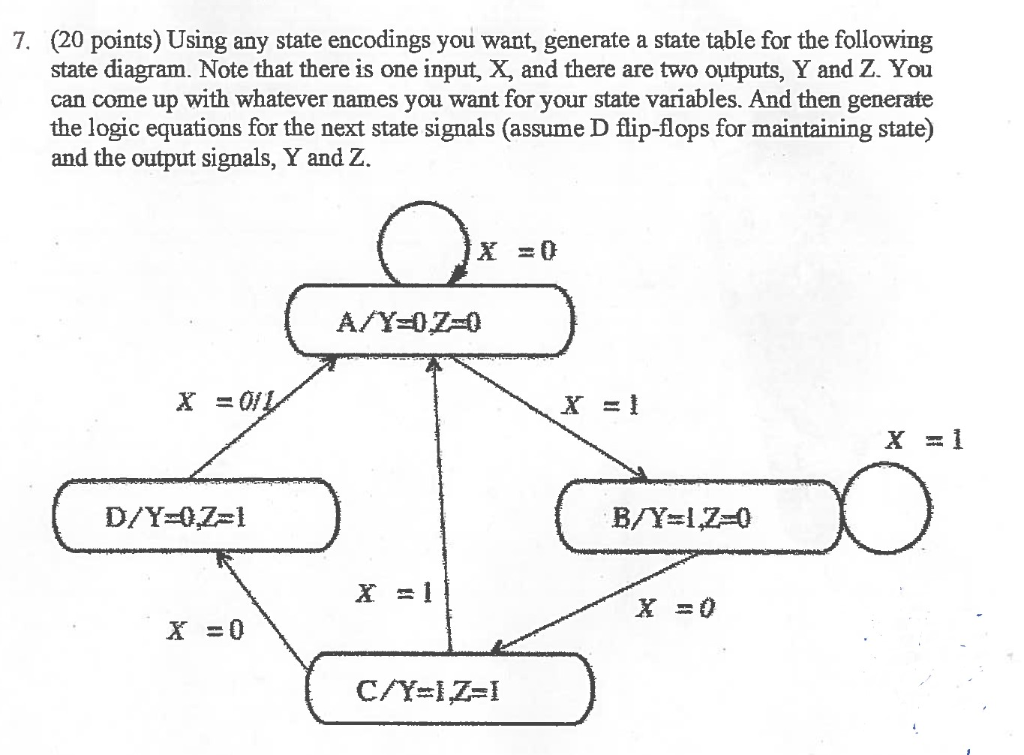(20 points) Using any state encodings you want, generate a state table for the following state diagram. Note that there is one input, X, and there are two outputs, Y and Z. You can come up with whatever names you want for your state variables. And then generate the logic equations for the next state signals (assume D flip-flops for maintaining state) and the output signals, Y and Z 7. A0 A/Y 070 x=1 x=1 x =1 x =0 x...

• ### Design a counter which counts from 12 up to 17 but skipping the value 13. When...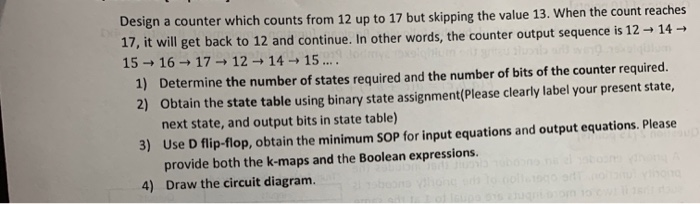Design a counter which counts from 12 up to 17 but skipping the value 13. When the count re aches 17, it will get back to 12 and continue. In other words, the counter output sequence is 12 14- 15 16-1712 14 15... Determine the number of states required and the number of bits of the counter required. 2) 1) Obtain the state table using binary state assignment(Please clearly label your present state, next state, and output bits in state...

• ### (a) Design an asynchronous Binary Coded Decimal (BCD) count-up counter using JK flip-flops. Draw the counter circuit clearly showing the configuration of the JK flip-flops and the necessary logic gat...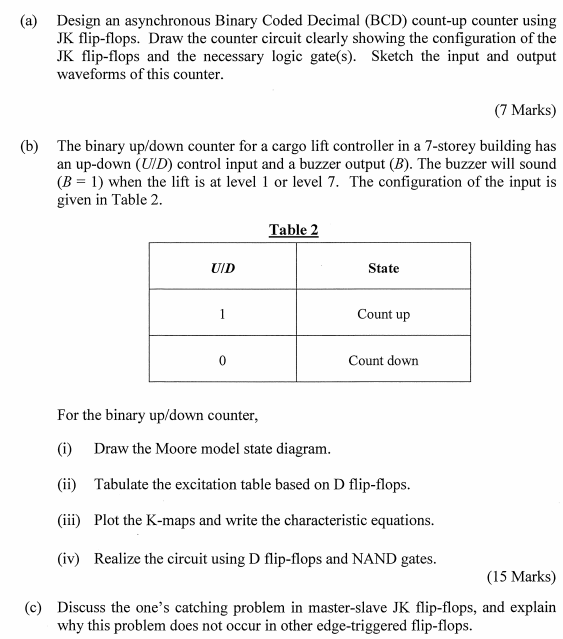(a) Design an asynchronous Binary Coded Decimal (BCD) count-up counter using JK flip-flops. Draw the counter circuit clearly showing the configuration of the JK flip-flops and the necessary logic gate(s). Sketch the input and output waveforms of this counter (7 Marks) (b) The binary up/down counter for a cargo lift controller in a 7-storey building has an up-down (UID) control input and a buzzer output (B). The buzzer will sound B 1) when the lift is at level 1 or...

• ### please, Teacher, help me with this question step by step please and explain everything, my Teacher?...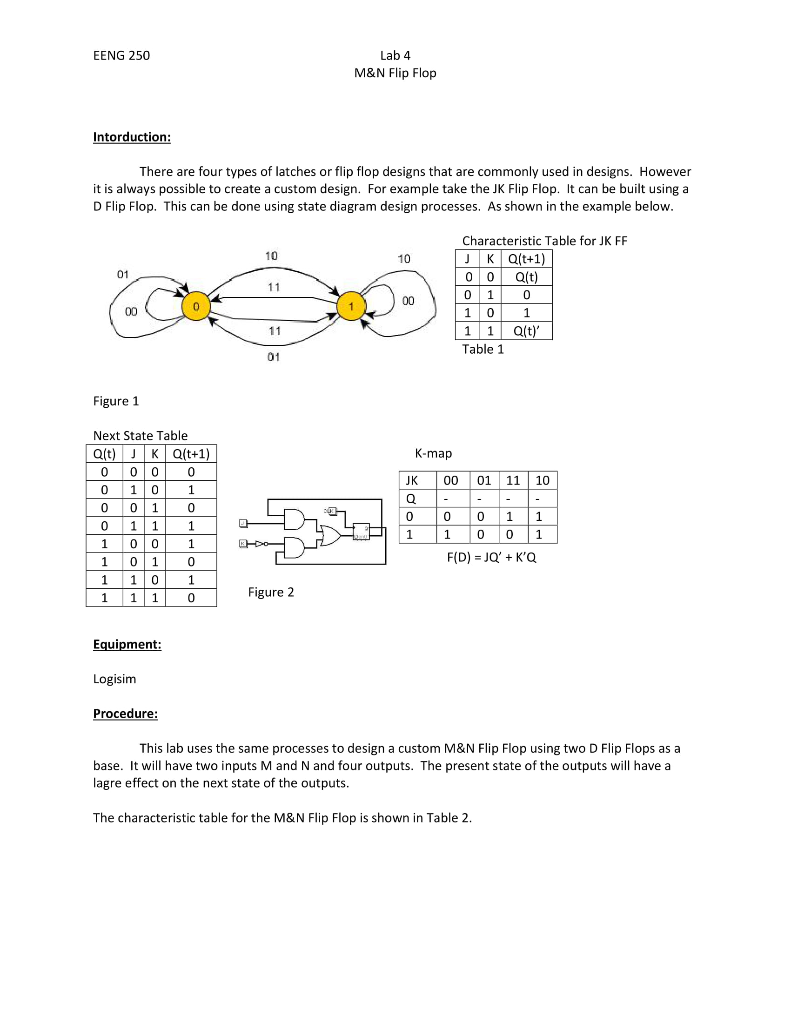please, Teacher, help me with this question step by step please and explain everything, my Teacher? EENG 250 Lab 4 M&N Flip Flop Intorduction: There are four types of latches or flip flop designs that are commonly used in designs. However it is always possible to create a custom design. For example take the JK Flip Flop. It can be built using a D Flip Flop. This can be done using state diagram design processes. As shown in the example...

• ### The task is to design a two-bit controlled counter which has two counting bits (Q2, Q1), has one control input C1, and a...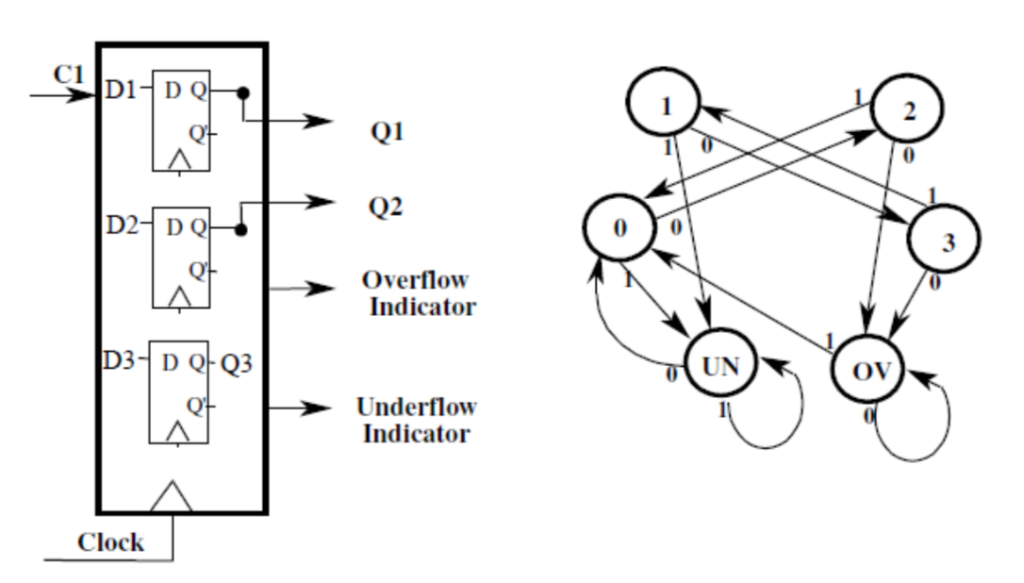The task is to design a two-bit controlled counter which has two counting bits (Q2, Q1), has one control input C1, and also two extra outputs, one indicating overflow, the other underflow. When C1=0 the counter counts up by 2s; i.e. 0 becomes 2, 1 becomes 3. In this mode the values 2 and 3 go to the overflow state. When the control input C1=1, the counter counts down by 2s, i.e. 3 becomes 1, and 2 becomes 0, and...

• ### In this lab, you will design a finite state machine to control the tail lights of...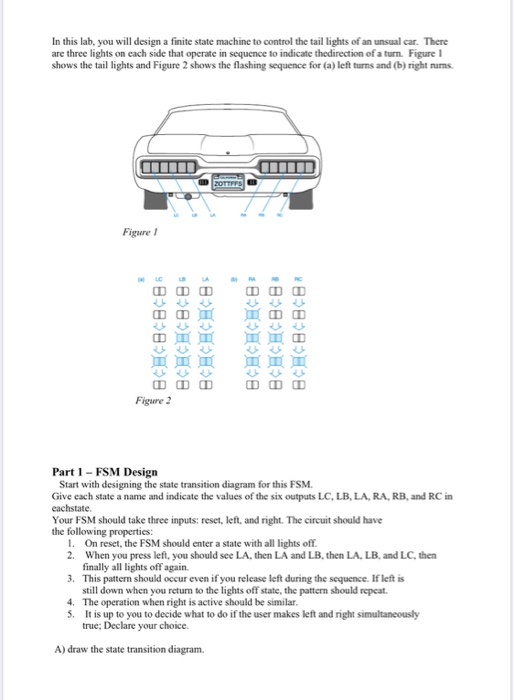In this lab, you will design a finite state machine to control the tail lights of an unsual car. There are three lights on each side that operate in sequence to indicate thedirection of a turn. Figure ! shows the tail lights and Figure 2 shows the flashing sequence for (a) left turns and (b) right rums. ZOTTAS Figure 28:8: BCECECece BCECECECes BCECECECB BCECECBCB 8888 Figure 2 Part 1 - FSM Design Start with designing the state transition diagram for...